# Hill Cipher

Hill cipher is a polygraphic substitution cipher based on linear algebra.Each letter is represented by a number modulo 26. Often the simple scheme A = 0, B = 1, …, Z = 25 is used, but this is not an essential feature of the cipher. To encrypt a message, each block of n letters (considered as an n-component vector) is multiplied by an invertible n × n matrix, against modulus 26. To decrypt the message, each block is multiplied by the inverse of the matrix used for encryption.

The matrix used for encryption is the cipher key, and it should be chosen randomly from the set of invertible n × n matrices (modulo 26).

Examples:

```Input  : Plaintext: ACT
Key: GYBNQKURP
Output : Ciphertext: POH
```
```Input  : Plaintext: GFG
Key: HILLMAGIC
Output : Ciphertext: SWK
```

Encryption

We have to encrypt the message ‘ACT’ (n=3).The key is ‘GYBNQKURP’ which can be written as the nxn matrix: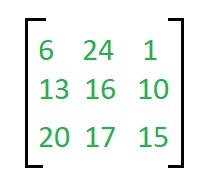The message ‘ACT’ is written as vector: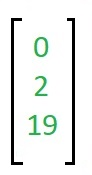The enciphered vector is given as: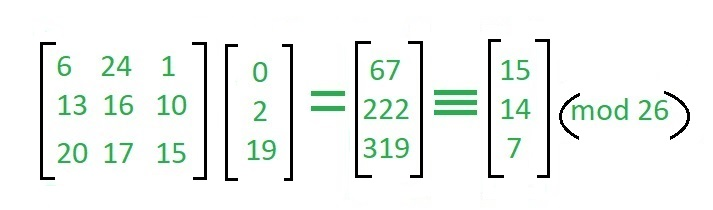which corresponds to ciphertext of ‘POH’

Decryption

To decrypt the message, we turn the ciphertext back into a vector, then simply multiply by the inverse matrix of the key matrix (IFKVIVVMI in letters).The inverse of the matrix used in the previous example is: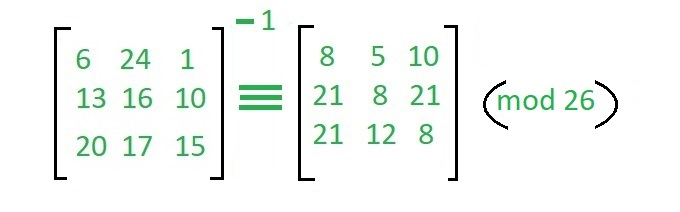For the previous Ciphertext ‘POH’: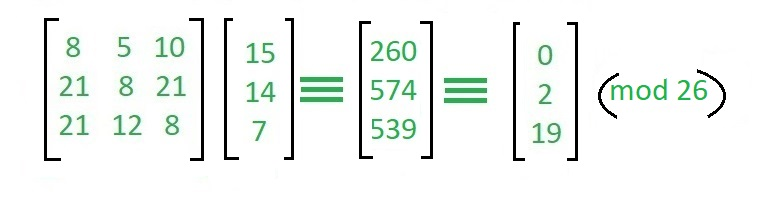which gives us back ‘ACT’.
Assume that all the alphabets are in upper case.
Below is the implementation of the above idea for n=3.

## C++

 `// C++ code to implement Hill Cipher ` `#include ` `using` `namespace` `std; ` ` `  `// Following function generates the ` `//  key matrix for the key string ` `void` `getKeyMatrix(string key, ``int` `keyMatrix[]) ` `{ ` `    ``int` `k = 0; ` `    ``for` `(``int` `i = 0; i < 3; i++)  ` `    ``{ ` `        ``for` `(``int` `j = 0; j < 3; j++)  ` `        ``{ ` `            ``keyMatrix[i][j] = (key[k]) % 65; ` `            ``k++; ` `        ``} ` `    ``} ` `} ` ` `  `// Following function encrypts the message ` `void` `encrypt(``int` `cipherMatrix[], ` `             ``int` `keyMatrix[],  ` `             ``int` `messageVector[]) ` `{ ` `    ``int` `x, i, j; ` `    ``for` `(i = 0; i < 3; i++)  ` `    ``{ ` `        ``for` `(j = 0; j < 1; j++) ` `        ``{ ` `            ``cipherMatrix[i][j] = 0; ` `          `  `             ``for` `(x = 0; x < 3; x++) ` `            ``{ ` `                ``cipherMatrix[i][j] +=  ` `                     ``keyMatrix[i][x] * messageVector[x][j]; ` `            ``} ` `         `  `            ``cipherMatrix[i][j] = cipherMatrix[i][j] % 26; ` `        ``} ` `    ``} ` `} ` ` `  `// Function to implement Hill Cipher ` `void` `HillCipher(string message, string key) ` `{ ` `    ``// Get key matrix from the key string ` `    ``int` `keyMatrix; ` `    ``getKeyMatrix(key, keyMatrix); ` ` `  `    ``int` `messageVector; ` ` `  `    ``// Generate vector for the message ` `    ``for` `(``int` `i = 0; i < 3; i++) ` `        ``messageVector[i] = (message[i]) % 65; ` ` `  `    ``int` `cipherMatrix; ` ` `  `    ``// Following function generates ` `    ``// the encrypted vector ` `    ``encrypt(cipherMatrix, keyMatrix, messageVector); ` ` `  `    ``string CipherText; ` ` `  `    ``// Generate the encrypted text from  ` `    ``// the encrypted vector ` `    ``for` `(``int` `i = 0; i < 3; i++) ` `        ``CipherText += cipherMatrix[i] + 65; ` ` `  `    ``// Finally print the ciphertext ` `    ``cout << ``" Ciphertext:"` `<< CipherText; ` `} ` ` `  `// Driver function for above code ` `int` `main() ` `{ ` `    ``// Get the message to be encrypted ` `    ``string message = ``"ACT"``; ` ` `  `    ``// Get the key ` `    ``string key = ``"GYBNQKURP"``; ` ` `  `    ``HillCipher(message, key); ` ` `  `    ``return` `0; ` `} `

## Java

 `// Java code to implement Hill Cipher ` `class` `GFG  ` `{ ` ` `  `// Following function generates the ` `// key matrix for the key string ` `static` `void` `getKeyMatrix(String key, ``int` `keyMatrix[][]) ` `{ ` `    ``int` `k = ``0``; ` `    ``for` `(``int` `i = ``0``; i < ``3``; i++)  ` `    ``{ ` `        ``for` `(``int` `j = ``0``; j < ``3``; j++)  ` `        ``{ ` `            ``keyMatrix[i][j] = (key.charAt(k)) % ``65``; ` `            ``k++; ` `        ``} ` `    ``} ` `} ` ` `  `// Following function encrypts the message ` `static` `void` `encrypt(``int` `cipherMatrix[][], ` `            ``int` `keyMatrix[][],  ` `            ``int` `messageVector[][]) ` `{ ` `    ``int` `x, i, j; ` `    ``for` `(i = ``0``; i < ``3``; i++)  ` `    ``{ ` `        ``for` `(j = ``0``; j < ``1``; j++) ` `        ``{ ` `            ``cipherMatrix[i][j] = ``0``; ` `         `  `            ``for` `(x = ``0``; x < ``3``; x++) ` `            ``{ ` `                ``cipherMatrix[i][j] +=  ` `                    ``keyMatrix[i][x] * messageVector[x][j]; ` `            ``} ` `         `  `            ``cipherMatrix[i][j] = cipherMatrix[i][j] % ``26``; ` `        ``} ` `    ``} ` `} ` ` `  `// Function to implement Hill Cipher ` `static` `void` `HillCipher(String message, String key) ` `{ ` `    ``// Get key matrix from the key string ` `    ``int` `[][]keyMatrix = ``new` `int``[``3``][``3``]; ` `    ``getKeyMatrix(key, keyMatrix); ` ` `  `    ``int` `[][]messageVector = ``new` `int``[``3``][``1``]; ` ` `  `    ``// Generate vector for the message ` `    ``for` `(``int` `i = ``0``; i < ``3``; i++) ` `        ``messageVector[i][``0``] = (message.charAt(i)) % ``65``; ` ` `  `    ``int` `[][]cipherMatrix = ``new` `int``[``3``][``1``]; ` ` `  `    ``// Following function generates ` `    ``// the encrypted vector ` `    ``encrypt(cipherMatrix, keyMatrix, messageVector); ` ` `  `    ``String CipherText=``""``; ` ` `  `    ``// Generate the encrypted text from  ` `    ``// the encrypted vector ` `    ``for` `(``int` `i = ``0``; i < ``3``; i++) ` `        ``CipherText += (``char``)(cipherMatrix[i][``0``] + ``65``); ` ` `  `    ``// Finally print the ciphertext ` `    ``System.out.print(``" Ciphertext:"` `+ CipherText); ` `} ` ` `  `// Driver code ` `public` `static` `void` `main(String[] args)  ` `{ ` `    ``// Get the message to be encrypted ` `    ``String message = ``"ACT"``; ` ` `  `    ``// Get the key ` `    ``String key = ``"GYBNQKURP"``; ` ` `  `    ``HillCipher(message, key); ` `    ``} ` `} ` ` `  `// This code has been contributed by 29AjayKumar `

## Python3

 `# Python3 code to implement Hill Cipher ` ` `  `keyMatrix ``=` `[[``0``] ``*` `3` `for` `i ``in` `range``(``3``)] ` ` `  `# Generate vector for the message ` `messageVector ``=` `[[``0``] ``for` `i ``in` `range``(``3``)] ` ` `  `# Generate vector for the cipher ` `cipherMatrix ``=` `[[``0``] ``for` `i ``in` `range``(``3``)] ` ` `  `# Following function generates the ` `# key matrix for the key string ` `def` `getKeyMatrix(key): ` `    ``k ``=` `0` `    ``for` `i ``in` `range``(``3``): ` `        ``for` `j ``in` `range``(``3``): ` `            ``keyMatrix[i][j] ``=` `ord``(key[k]) ``%` `65` `            ``k ``+``=` `1` ` `  `# Following function encrypts the message ` `def` `encrypt(messageVector): ` `    ``for` `i ``in` `range``(``3``): ` `        ``for` `j ``in` `range``(``1``): ` `            ``cipherMatrix[i][j] ``=` `0` `            ``for` `x ``in` `range``(``3``): ` `                ``cipherMatrix[i][j] ``+``=` `(keyMatrix[i][x] ``*`  `                                       ``messageVector[x][j]) ` `            ``cipherMatrix[i][j] ``=` `cipherMatrix[i][j] ``%` `26` ` `  `def` `HillCipher(message, key): ` ` `  `    ``# Get key matrix from the key string ` `    ``getKeyMatrix(key) ` ` `  `    ``# Generate vector for the message ` `    ``for` `i ``in` `range``(``3``): ` `        ``messageVector[i][``0``] ``=` `ord``(message[i]) ``%` `65` ` `  `    ``# Following function generates ` `    ``# the encrypted vector ` `    ``encrypt(messageVector) ` ` `  `    ``# Generate the encrypted text  ` `    ``# from the encrypted vector ` `    ``CipherText ``=` `[] ` `    ``for` `i ``in` `range``(``3``): ` `        ``CipherText.append(``chr``(cipherMatrix[i][``0``] ``+` `65``)) ` ` `  `    ``# Finally print the ciphertext ` `    ``print``(``"Ciphertext: "``, "".join(CipherText)) ` ` `  `# Driver Code ` `def` `main(): ` ` `  `    ``# Get the message to  ` `    ``# be encrypted ` `    ``message ``=` `"ACT"` ` `  `    ``# Get the key ` `    ``key ``=` `"GYBNQKURP"` ` `  `    ``HillCipher(message, key) ` ` `  `if` `__name__ ``=``=` `"__main__"``: ` `    ``main() ` ` `  `# This code is contributed  ` `# by Pratik Somwanshi `

## C#

 `// C# code to implement Hill Cipher ` `using` `System; ` ` `  `class` `GFG  ` `{  ` ` `  `// Following function generates the  ` `// key matrix for the key string  ` `static` `void` `getKeyMatrix(String key,  ` `                         ``int` `[,]keyMatrix)  ` `{  ` `    ``int` `k = 0;  ` `    ``for` `(``int` `i = 0; i < 3; i++)  ` `    ``{  ` `        ``for` `(``int` `j = 0; j < 3; j++)  ` `        ``{  ` `            ``keyMatrix[i, j] = (key[k]) % 65;  ` `            ``k++;  ` `        ``}  ` `    ``}  ` `}  ` ` `  `// Following function encrypts the message  ` `static` `void` `encrypt(``int` `[,]cipherMatrix,  ` `                    ``int` `[,]keyMatrix,  ` `                    ``int` `[,]messageVector)  ` `{  ` `    ``int` `x, i, j;  ` `    ``for` `(i = 0; i < 3; i++)  ` `    ``{  ` `        ``for` `(j = 0; j < 1; j++)  ` `        ``{  ` `            ``cipherMatrix[i, j] = 0;  ` `         `  `            ``for` `(x = 0; x < 3; x++)  ` `            ``{  ` `                ``cipherMatrix[i, j] += keyMatrix[i, x] *  ` `                                      ``messageVector[x, j];  ` `            ``}  ` `         `  `            ``cipherMatrix[i, j] = cipherMatrix[i, j] % 26;  ` `        ``}  ` `    ``}  ` `}  ` ` `  `// Function to implement Hill Cipher  ` `static` `void` `HillCipher(String message, String key)  ` `{  ` `     `  `    ``// Get key matrix from the key string  ` `    ``int` `[,]keyMatrix = ``new` `int``[3, 3];  ` `    ``getKeyMatrix(key, keyMatrix);  ` ` `  `    ``int` `[,]messageVector = ``new` `int``[3, 1];  ` ` `  `    ``// Generate vector for the message  ` `    ``for` `(``int` `i = 0; i < 3; i++)  ` `        ``messageVector[i, 0] = (message[i]) % 65;  ` ` `  `    ``int` `[,]cipherMatrix = ``new` `int``[3, 1];  ` ` `  `    ``// Following function generates  ` `    ``// the encrypted vector  ` `    ``encrypt(cipherMatrix, keyMatrix, messageVector);  ` ` `  `    ``String CipherText = ``""``;  ` ` `  `    ``// Generate the encrypted text from  ` `    ``// the encrypted vector  ` `    ``for` `(``int` `i = 0; i < 3; i++)  ` `        ``CipherText += (``char``)(cipherMatrix[i, 0] + 65);  ` ` `  `    ``// Finally print the ciphertext  ` `    ``Console.Write(``"Ciphertext: "` `+ CipherText);  ` `}  ` ` `  `// Driver code  ` `public` `static` `void` `Main(String[] args)  ` `{  ` `    ``// Get the message to be encrypted  ` `    ``String message = ``"ACT"``;  ` ` `  `    ``// Get the key  ` `    ``String key = ``"GYBNQKURP"``;  ` ` `  `    ``HillCipher(message, key);  ` `}  ` `}  ` ` `  `// This code is contributed by Rajput-Ji  `

Output:

```Ciphertext: POH
```

In a similar way you can write the code for decrypting the encrypted message by following the steps explained above.
Reference: https://en.wikipedia.org/wiki/Hill_cipher

Attention reader! Don’t stop learning now. Get hold of all the important DSA concepts with the DSA Self Paced Course at a student-friendly price and become industry ready.

My Personal Notes arrow_drop_upCheck out this Author's contributed articles.

If you like GeeksforGeeks and would like to contribute, you can also write an article using contribute.geeksforgeeks.org or mail your article to contribute@geeksforgeeks.org. See your article appearing on the GeeksforGeeks main page and help other Geeks.

Please Improve this article if you find anything incorrect by clicking on the "Improve Article" button below.